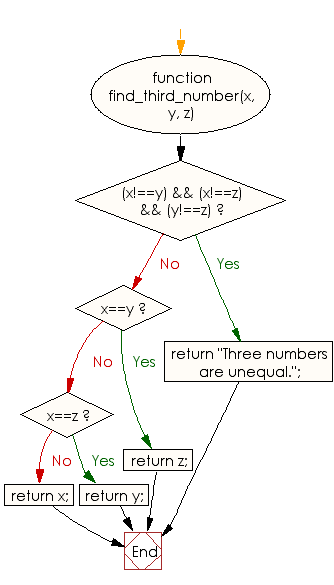# JavaScript: Check a number from three given numbers where two numbers are equal, find the third one

## JavaScript Basic: Exercise-111 with Solution

Write a JavaScript program to check a number from three given numbers where two numbers are equal, find the third one.

Pictorial Presentation:Sample Solution:

HTML Code:

``````<!DOCTYPE html>
<html>
<meta charset="utf-8">
<meta name="viewport" content="width=device-width">
<title> Check a number from three given numbers where two numbers are equal, find the third one</title>
<body>

</body>
</html>
```
```

JavaScript Code:

``````function find_third_number(x, y, z) {
if((x!==y) && (x!==z) && (y!==z))
return "Three numbers are unequal.";
if(x==y) return z;
if(x==z) return y;
return x;
}

console.log(find_third_number(1,2,2))
console.log(find_third_number(1,1,2))
console.log(find_third_number(1,2,3))
``````

Sample Output:

```1
2
Three numbers are unequal.
```

Flowchart:ES6 Version:

``````function find_third_number(x, y, z) {
if((x!==y) && (x!==z) && (y!==z))
return "Three numbers are unequal.";
if(x==y) return z;
if(x==z) return y;
return x;
}

console.log(find_third_number(1,2,2))
console.log(find_third_number(1,1,2))
console.log(find_third_number(1,2,3))
``````

Live Demo:

See the Pen javascript-basic-exercise-111 by w3resource (@w3resource) on CodePen.

What is the difficulty level of this exercise?

Test your Programming skills with w3resource's quiz.

﻿

## JavaScript: Tips of the Day

Checks if a string is an anagram of another string (case-insensitive, ignores spaces, punctuation and special characters)

Example:

```const isAnagram = (str1, str2) => {
const normalize = str =>
str
.toLowerCase()
.replace(/[^a-z0-9]/gi, '')
.split('')
.sort()
.join('');
return normalize(str1) === normalize(str2);
};
console.log(isAnagram('iceman', 'cinema')); // true
```

Output:

```true
```